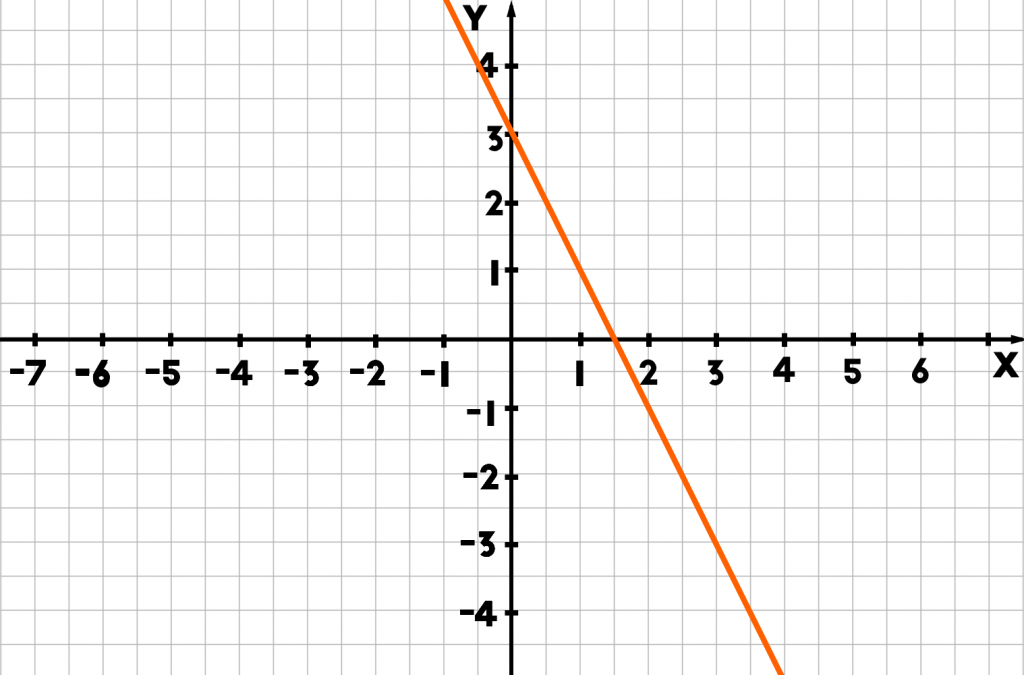# Math Test

Name:

1) Which of these numbers does this symbol represent:

### π

3.15
3.14159
3.41159
3.14195

2) Which of these mathematical equations are true:

√10 < π
√10 > π
√10 ≈ π
√10 = π

3) In what orientation is this line:

### y=¾x–3

Postitive
Negative
Horizontal
Vertical;

4) What is the equation for this line in slope-intercept form: